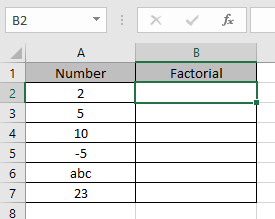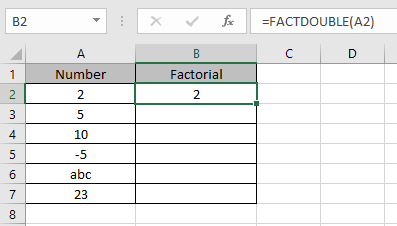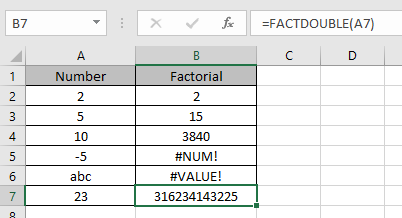# How to use the FACTDOUBLE Function in Excel

In this article, we will learn about how to use the FACTDOUBLE function in Excel. FACTDOUBLE function is a mathematical operator used to get the double Factorial of a number. FACTDOUBLE of a number (n) is calculated using the below show formula. N (even) = 2 x 4 x 6 x 8 x …. x (n-2) x n N (odd) = 1 x 3 x 5 x 7 x …. x (n-2) x n The FACTDOUBLE function returns the double Factorial of the input number. FACTDOUBLE function takes the product of alternate numbers and goes up to value n Syntax:

=FACTDOUBLE (number)

Let’s understand this function using it in an example.Here we have some values which we will be providing to the function and to see what the function returns. Use the formula:

=FACTDOUBLE(A2)

A2 : number Here argument to the formula is taken as cell reference.As you can see the formula returns the double Factorial of the number 2 is 2. Now copy the formula in other cells using Ctrl + D shortcut key.These are the results returned by the FACTDOUBLE function.

Use FACTDOUBLE function with other function to get results. Notes:

1. The function returns #NUM! Error, if the argument to the function is negative numbers or text without using quotes.
2. The function returns #VALUE! Error, if the argument to the function is text with quotes or text given in a cell reference.
3. If the number is in decimal then excel truncates it to integer and then return the value

Hope you understood how to use FACTDOUBLE function and referring cell in Excel. Explore more articles on Excel function here. Please feel free to state your query or feedback for the above article.

Related Article:

How to use the FACT Function in Excel

How to use the Excel POWER function

Popular Articles:

50 Excel Shortcuts to Increase Your Productivity

How to use the VLOOKUP Function in Excel

How to use the COUNTIF in Excel 2016

How to use the SUMIF Function in Excel

Terms and Conditions of use

The applications/code on this site are distributed as is and without warranties or liability. In no event shall the owner of the copyrights, or the authors of the applications/code be liable for any loss of profit, any problems or any damage resulting from the use or evaluation of the applications/code.